# Perfect number

This article defines a property that can be evaluated for a natural number, i.e., every natural number either satisfies the property or does not satisfy the property.
View a complete list of properties of natural numbers

## Definition

A natural numberis termed a perfect number if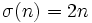, where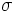denotes the divisor sum function: the sum of all the positive divisors of. In particular,equals the sum of all its proper positive divisors.

## Relation with other properties

### Variations

• Almost perfect number: This requires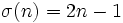.
• Quasiperfect number: This requires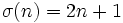.

### Opposite properties

• Abundant number: This requires.
• Deficient number: This requires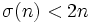.

## Facts

• If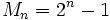(theMersenne number) is a prime number (and hence, a Mersenne prime), then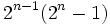is a perfect number.
• Every even perfect number arises in the above fashion.
• The existence of odd perfect numbers is an open problem.